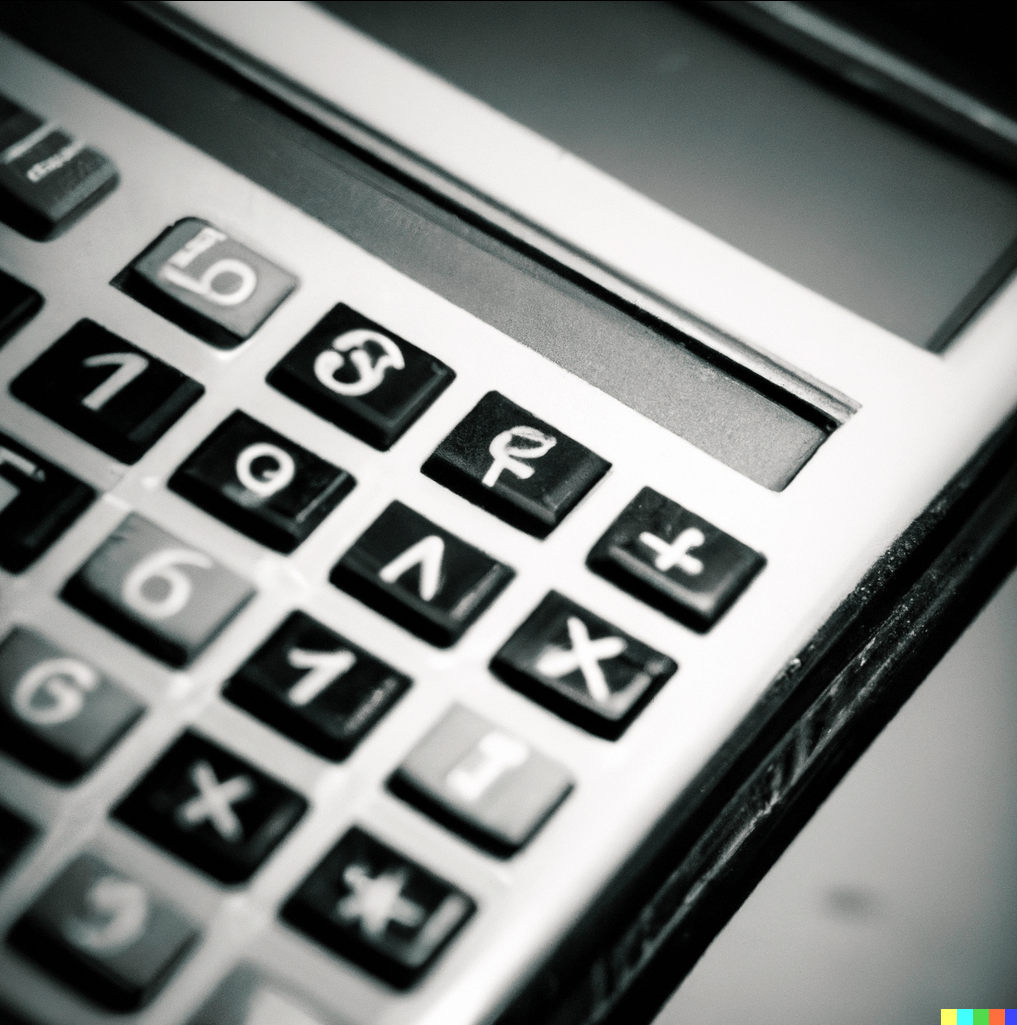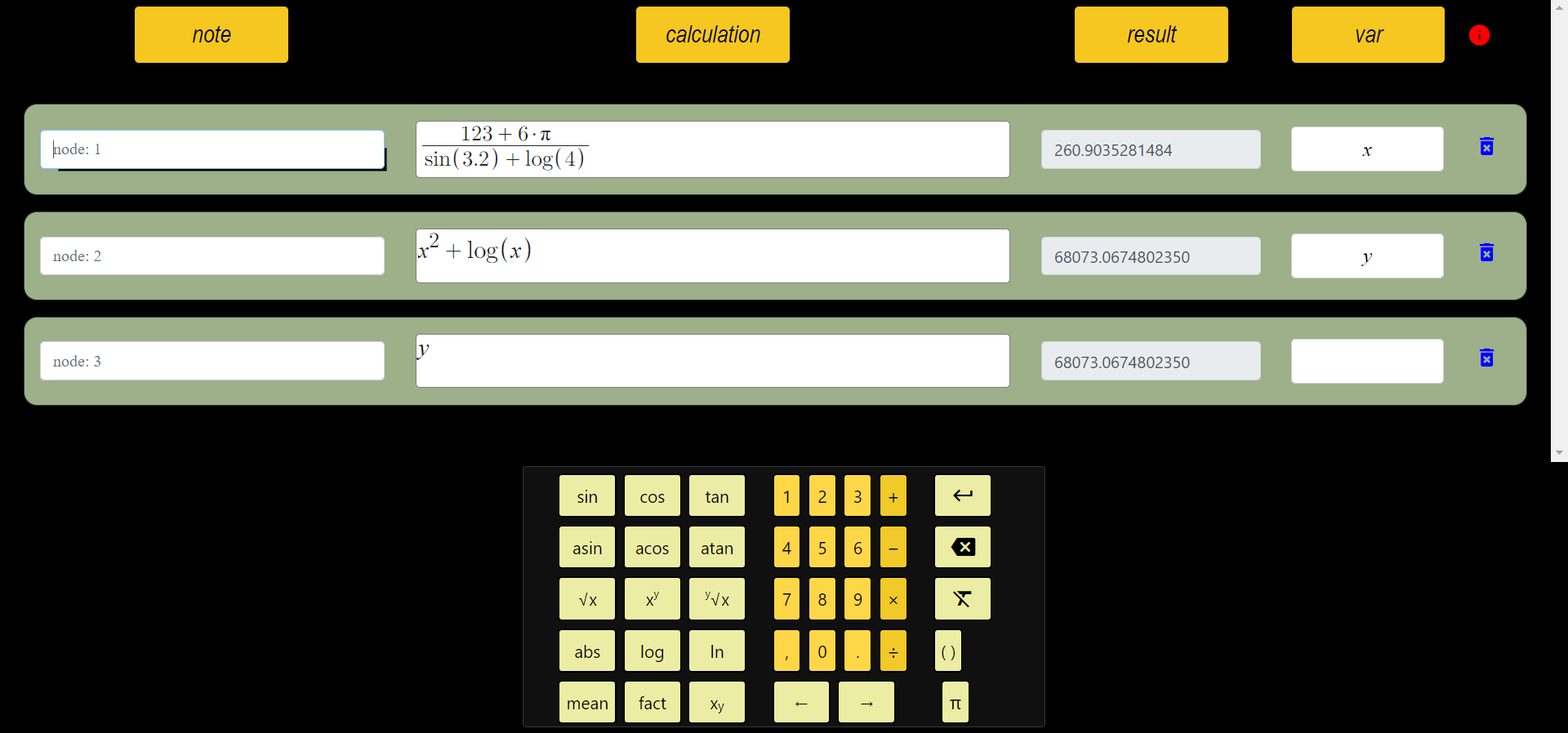# What is Calculator Software? Where is it used?

Calculator software is a program used to perform mathematical calculations. It generally functions the same as calculators used for simple calculations. Calculator software can be found on devices such as computers, phones, tablets and is compatible with many different operating systems.Besides basic mathematical operations, calculator software can include many different functions such as trigonometry, logarithms, square roots, exponentiation, and more complex mathematical operations. Some calculator software may also include features such as unit conversion, interest calculation, tax calculation, and currency conversion.

Calculator software usually has a simple user interface and is easy to use. Some calculator software is available in many different languages and may run in a user-preferred language.

Calculator software is a useful tool in engineering, finance, mathematics and many other fields and can be designed to meet the needs of users of all levels.

## History

Although it does not have a specific history, calculator software started to become widespread with the invention of computers. While the calculator software that came with the first personal computers had very simple functions, calculator software with more complex functions started to be produced over time.

In the 1980s, calculator software that came with the first computer operating systems could usually only do four operations. However, over time, the functions of these software have increased and along with mathematical operations such as trigonometry, logarithm, exponentiation, square root, functions such as unit conversion, currency conversion, tax calculation and interest calculation have been added.

LEO (Lyons Electronic Office) was founded in 1951 in England by J. Lyons & Co. It is considered to be the first commercial computer developed by LEO did not have the features of today's computers, and it did not have features similar to the calculators used today.

The development of LEO led to the proliferation of automated computing systems in the business world. LEO could be used for a variety of transactions, such as the company's orders, inventories, and financial transactions. Therefore, the development of LEO has meant great progress and modernization for businesses.

## VisiCalc

VisiCalc was the first spreadsheet program developed by Dan Bricklin and Bob Frankston in 1979. VisiCalc has contributed to the widespread commercial use of personal computers and has been widely used in many fields, especially in finance, accounting and business.

VisiCalc has replaced previously manual calculations, making calculations faster and easier. The program was designed in a spreadsheet format that allowed multiple calculations to be performed on a single page. This allowed users to automatically recalculate when users changed the data in one cell, other related cells.

VisiCalc was designed for use on personal computers such as the Apple II, helping businesses cut costs and perform financial analysis more quickly. In addition, VisiCalc formed the basis of later spreadsheet programs and has played a major role in the development of many spreadsheet programs today, such as Excel.

## Visual Computing SoftwareThere are many software available for visual calculations. Some popular software are:

### MATLAB

Mathematical is a widely used software for the creation of graphs. Supports 2D and 3D graphs.

### Mathematica

Mathematical calculation and visualization software. It's pretty good at creating mathematical graphs of functionsgraphs of functions (2D and 3D).

### Python matplotlib

It is a plotting graphs library for the Python programming language. It supports 3D graphics as well as 2D graphics.

### Maple

It is scientific computing software. It can be used to create 2D and 3D graphs of mathematical functions, as well as to analyze mathematical models.

###### All Articles

id: ezjtUrGOl2